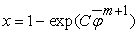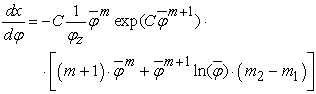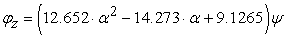﻿ Analyze and Mathematical Modeling of the Combustion Process of One-Cylinder Spark Ignited Hydrogen Fueled Engine

### Analyze and Mathematical Modeling of the Combustion Process of One-Cylinder Spark Ignited Hydrogen F...

Rachid Maamri, Alexandre Petrovitsh Martshenko, O. O. Osetrov, Yves Dubé, Lotfi Toubal, Agbossou KodjoOPEN ACCESSPEER-REVIEWED

## Analyze and Mathematical Modeling of the Combustion Process of One-Cylinder Spark Ignited Hydrogen Fueled Engine

Rachid Maamri1,, Alexandre Petrovitsh Martshenko2, O. O. Osetrov2, Yves Dubé1, Lotfi Toubal1, Agbossou Kodjo1

1Department of Mechanical Engineering, Trois Rivières University in Quebec, Trois Rivières, Canada

2Department of Internal Combustion Engines, Kharkov National Technical University, Kharkov, Ukraine

### Abstract

Hydrogen is a potential replacement for fossil fuels in the transportation sector because it is clean, renewable and has the possibility to reduce emission of criteria pollutants (NOx) and the effect on global warming via carbon dioxide which is negligible. The present research study is devoted to analyze the process of combustion of hydrogen in the four-cycle one-cylinder spark ignited engine and to concern with developing a numerical model that consider the features of process of combustion, to estimate the influence of the constructive and the control parameters of the engine on process of combustion. It is found that the curve of speed of combustion at hydrogen blending is close to the triangular form. It was found that varying the compression ratio and, to a lesser extent, the ignition timing influences the duration and the dynamics of the combustion process, more than at varying the air–fuel ratio.

### At a glance: Figures

12
Prev Next

• Maamri, Rachid, et al. "Analyze and Mathematical Modeling of the Combustion Process of One-Cylinder Spark Ignited Hydrogen Fueled Engine." American Journal of Vehicle Design 1.2 (2013): 21-24.
• Maamri, R. , Martshenko, A. P. , Osetrov, O. O. , Dubé, Y. , Toubal, L. , & Kodjo, A. (2013). Analyze and Mathematical Modeling of the Combustion Process of One-Cylinder Spark Ignited Hydrogen Fueled Engine. American Journal of Vehicle Design, 1(2), 21-24.
• Maamri, Rachid, Alexandre Petrovitsh Martshenko, O. O. Osetrov, Yves Dubé, Lotfi Toubal, and Agbossou Kodjo. "Analyze and Mathematical Modeling of the Combustion Process of One-Cylinder Spark Ignited Hydrogen Fueled Engine." American Journal of Vehicle Design 1, no. 2 (2013): 21-24.

 Import into BibTeX Import into EndNote Import into RefMan Import into RefWorks

### 1. Introduction

Hydrogen is considered as a fuel perspective view, including for internal combustion engines. First, at its burning practically there are no emissions of CO, СН and solid particles. Their some presence in the exhaust gases speaks burning out of lubricant oil. Secondly, hydrogen wide flammability limits allow organising qualitative regulation of capacity on operational modes, and, as consequence, to increase engine efficiency on part loads. Thirdly, at burning of poor hydrogen/air mixtures, it is possible to lower considerably NO emissions essential material resources. To hydrogen lacks carry the high cost price of its production, difficulty of storage of hydrogen onboard a vehicle, absence of an infrastructure, etc.

Advantages of hydrogen cause development of the works connected with use of given fuel in internal combustion engines [1-7].

It is known that one of the most effective tools of research of processes in internal combustion engine and optimisation of its design is mathematical modelling. By working out of mathematical models there is some question, not shined widely enough in the technical literature.

It concerns dynamics of a thermal emission in the hydrogen engine and the influence on working process of constructive and adjusting parameters of the engine.

### 2. Experimental Setup

Results of the experiments performed on one-cylinder four-cycle CFR engine by the Belgian scientists  are put in a work basis. Relevant engine characteristics are shown in Table 1, the schematic view of the test equipments is shown in Figure 1.

#### Table 1. Relevant engine characteristicsDownload asPowerPoint Slide

Veiw figureView current table in a new window

CFR engine intended originally for definition of combustible octane number has been converted for hydrogen use. On the engine have established a hydrogen injection system in the inlet manifold. The quantity of submitted fuel changed regulation of duration of the valve lift upon the injector nozzle.

In a cylinder head the piezoelectric sensor cooled by water for pressure measurement has been established. Also loads, frequency of rotation of a cranked shaft, TDC position, mass consumption of air and hydrogen, mixture pressure in front of valves and exhaust gases pressure were measured. In the exhaust gases the concentration of oxygen О2, hydrogen Н2, nitrogen oxides NO, nitrogen dioxide NO2, carbon monoxide CO, carbon dioxide СО2 and unburned hydrocarbons СН was defined. The frequency of rotation in all tests was supported by a constant n = 600 min-1 and the compression ratio changed in a range from 7 to 9.5.

The experimental data received on CFR engine, have allowed to specify model of combustion of hydrogen in the four-cycle engine with spark ignition.

### 3. Results and Discussion

3.1. Analysis and Mathematical Modelling of Process of Hydrogen Combustion

Now are known single, two and multi-zones combustion models. Laws of mass and energy conservation are put in a basis single-zone models. Quantity of the burned fuel by time and speed of the heat release are set by empirical or semi-empirical dependences (for example, Wiebe's technique). The co-ordinate of time is introduced.

In multi-zones models of the equation of laws of mass and energy conservation in the differential form are supplemented with the equations of Nave-Stocks describing turbulent flow of gas, and, besides time, spatial co-ordinates are entered.

In two-zone models the spatial co-ordinate is the radius of front of a flame. Combustion process is modelled on the basis of the data about speed of propagation of a flame in laminar and turbulent environments.

The inconvenient of two- and multi-zone models is the necessity and the complexity of the use of a considerable quantity of the boundary conditions received as a result of experimental or theoretical researches. Speed of propagation of a flame, as a rule, is set by empirical formulas on the basis of experimental data about speed of laminar and turbulent burning in bombs [2, 4, 5, 8, 9]. The account of specificity of combustion in internal combustion engine is made by empirical factors that reduce accuracy of calculations at researches for other types of engines. The field of application of the given models – detailed theoretical study of combustion.

In the present work for research and modelling of process of combustion of hydrogen the thermodynamic model used is Wiebe based. This single-zone semi-empirical model calculates the rate of mass that is burned and the speed of combustion are put:(1)(2)

where, φ, φz - accordingly relative time of combustion, current time from the beginning of combustion and burn duration presented in angles of rotation of a crankshaft; C – a constant (for example, the constant C = ln (1-0.999) = -6.908 corresponds to a combustion efficiency of 99.9 %); m - Wiebe exponent or indicator of character of combustion – a constant defining dynamics of process of combustion. Constants φz and m in the given model are selected by an empirical method from a condition of providing the similarity between calculated and measured pressure-crank angle diagrams (the combustion law). As a rule, researchers define dependences φz and m from constructive and regime parameters of working process, and then use the given dependences in optimisation and in other works. In this work, we have to define the influence of constructive and regime parameters of working process on the law of combustion and, accordingly, parameters φz and m Wiebe's models.

On Figure 2, the influence of burn duration φz and the Wiebe exponent m on the form of the pressure-crank angle diagram, are shown.

Figure 2. Selection of Wiebe exponent m (a) and burn duration φz (b) in formulas (1) and (2) for a regime when n = 600 min-1 and Ne = 1.3 kW. By points the measured diagram is shown

It is visible that for a regime when n = 600 min-1 and Ne = 1.3 kW, the best similarity between calculated and measured diagrams is reached at use of φz= 22° crank angle and m = 2.75. However and in this case the inclination of calculated and measured curves of pressure does not coincide. It is obvious that combustion occurs more intensively in an initial phase and less intensively in a final phase, that is to say the combustion law, is close to the triangular form (Figure 3). We offer a variable Wiebe exponent, changing on linear dependence from m1 = 1.5 to m2 = 4:(3)

In this case the speed of combustion is defined under the formula:(4)
Figure 3. Combustion speed (а) and pressure- crank angle diagram (b) with Wiebe exponent m. Burn duration φz = 22°crank angle. By points the CFR engine experimental diagram is shown. n = 600 min-1, Ne =1.3 kW

This figure shows, that the use of a variable Wiebe exponent m provide a good similarity between measured and calculated pressure-crank angle diagrams for the regime with n = 600 min-1 and Ne = 1.3 kW.

Figure 4. Influence of a spark advance θоп (a), compression ratio ε (b) and air–fuel excess ratio α (c,d) on measured and calculated pressure-crank angle diagrams. By points experimental diagrams are shown

Figure 4 shows the influence of the spark advance θоп, the compression ratio ε and the air–fuel excess ratio α on measured and calculated pressure-crank angle diagrams. At the time of the modelling of Wiebe exponent, we put linear dependence (3), where coefficients m1 and m2 don’t change and we take the combustion duration φz as a constant equal to 22° crank angle (Figure 4. а-c).

We observe that the variation of the spark advance and the compression ratio of the engine, don’t have any influence on the dynamics and on the hydrogen burn duration. In the same time, the influence of the air–fuel excess ratio on the burn duration is considerable (Figure 4. c). This fact confirms measurements.

We found an empirical relation between φz and α: for the regime with n = 600 min-1 and Ne = 1.3 kW:(5)

where ψ – coefficient, defining the influence of the turbulence and other factors on combustion duration.

The use of this relation allows a good similarity between measurements and calculations (Figure 4. d).

The relation (5) allows an acceptable similarity between calculated and measured results for CFR engine only for regimes with n=600 min-1. Obviously, for the regimes with wide crank angle, the burn duration decreases because the in-cylinder mixture turbulence increases. Moreover, for engines with other dimension and a different configuration of the inlet manifold, the in-cylinder turbulence will change. Consequently, the coefficient ψ must be reduced or increased.

### 4. Conclusions

As a result of theoretical researches it is established that the form of curve speed of combustion at hydrogen use is close to the triangular.

It is offered to use in the model of combustion of Wiebe a variable indicator of character of combustion m (Wiebe exponent), changing on the linear law that allows to describe dynamics of combustion of hydrogen more precisely.

It is shown that varying the compression ratio and, to a lesser extent, the ignition timing influences the duration and the dynamics of the combustion process, more than at varying the air–fuel ratio. To consider this influence use of approximating dependence of duration of combustion allows φz from air–fuel excess ratio α.

### References

  Mishenko A.I. Application of hydrogen for automobile engines / A.I.Mishenko. - Kiev: Sciences. Dumka, 1984.-143 p.In article  Verhelst S. A study of the combustion in hydrogen-fuelled internal combustion engines / Verhelst S.//Department of Flow, Heat and Combustion Mechanics, Ghent University, 2005.-222 p.In article  Kim J.M. Performance characteristics of hydrogen fueled engine with the direct injection and spark ignition system / J.M. Kim, Y.T. Kim, J.T. Lee, S.Y. Lee//SAE. – Paper N° 95249.-1995.In article  Polasek M. Application of advanced simulation methods and their combination with experiments to modelling of hydrogen fueled engine emission potentials / M. Polasek, J. Macek, M.Takats, O. Vıtek//SAE. – Paper N° 2002-01-0373.-2002.In article  Ma J. Simulation and prediction on the performance of a vehicle’s hydrogen engine / J. Ma, Y. Su, Y. Zhou, Z. Zhang//Int. J. Hydrogen Energy, 28:77-83, 2003.In article CrossRef  Sierens R. Experimental study of a hydrogen fuelled engine. Proceedings ASME Spring Engine Technology Conference / R Sierens, S. Verhelst//ICE 34-3. – Paper N °2000-ICE-285. – pp. 9-16. – San Antonio.-2000.In article  Kobayashi H. and Kawazoe H. Flame instability effects on the smallest wrinkling scale and burning velocity of high pressure turbulent premixed flames. 28th Symposium (International) on Combustion, pages 375-382, 2000.In article  Lipatnikov A.N. Turbulent flame speed and thickness: phenomenology, evaluation, and application in multidimensional simulations / A.N. Lipatnikov, J. Chomiak//Progress in Energy and Combustion Science. – 28:1-74.-2002.In article  Keck J.C. Early flame development and burning rates in spark ignition engines and their cyclic variability/J.C. Keck, Heywood J.B., and Noske G. //SAE. – Paper N°870164.-1987.In article